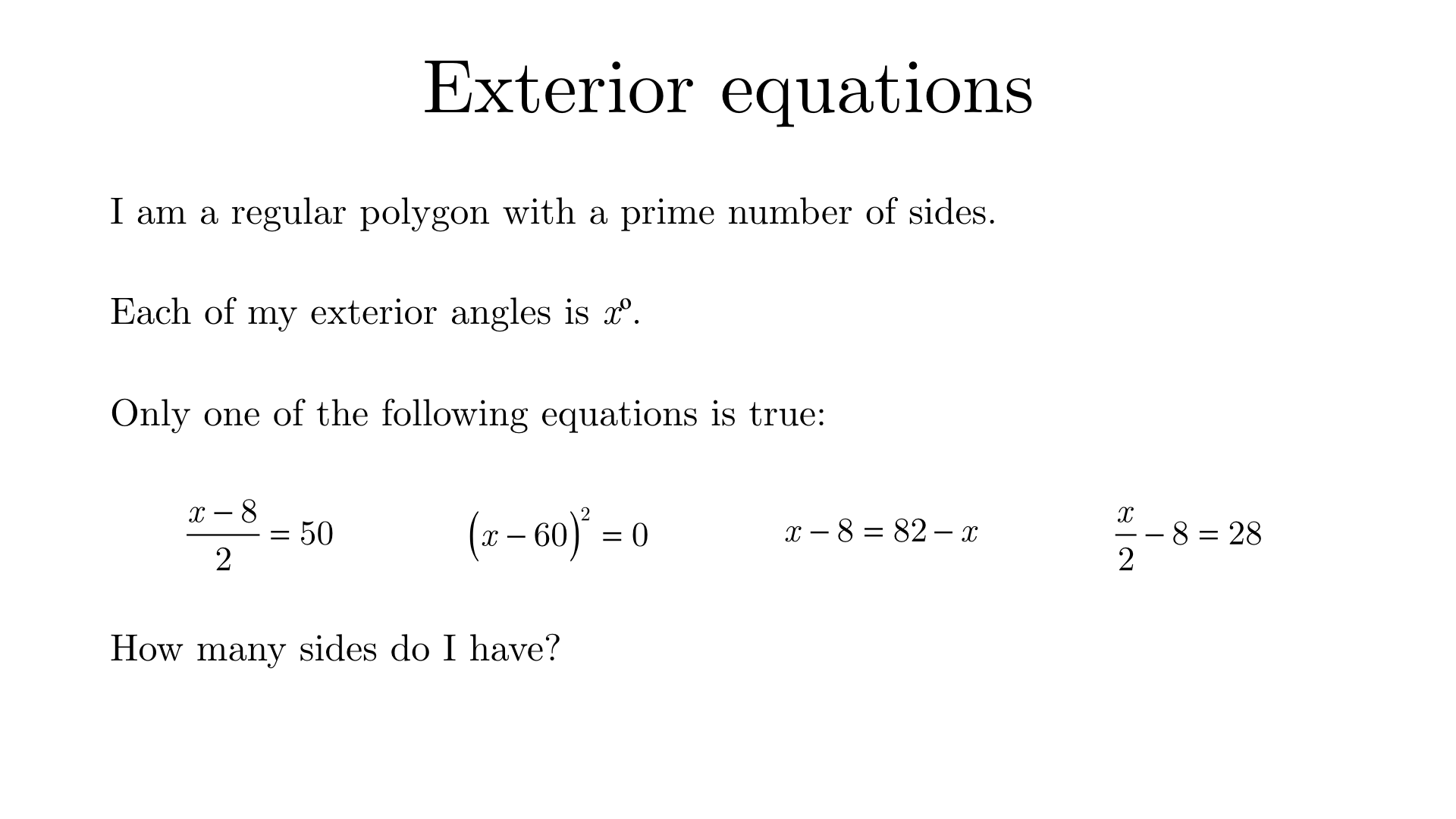Solution

Solving each the equations, we find that either $$x = 108$$, $$x = 60$$, $$x = 45$$, or $$x = 72$$.

The exterior angle of a regular polygon must be factor of 360, so this rules out $$x = 108$$.

For the remaining three cases, $$60$$º is the exterior angle for regular hexagons, $$45$$º is for regular octagons, and $$72$$º is for regular pentagons.

Only the pentagon has a prime number of sides. Therefore, the polygon has 5 sides.